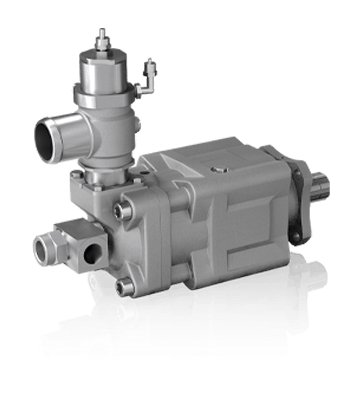# SLPD 20/20-64/32 DIN SAVTEC

SLPD 20/20-64/32 DIN Savtec is equipped with a shut-off valve, this makes it possible to control so that oil only feeds when required.

SLPD 20/20-64/32 DIN Savtec´s valve is available as pneumatic remote control. The signal is obtained from a panel switch or automatically from, e.g. the parking brake, pressure sensor or diode gate.### Product BenefitsIndependent direction of rotationA cost effective total solution in relation to a conventional installation using two pumps in a splitter gear boxSmooth operation over the entire speed rangeLong life due to high demands on material selection, such as bearings, seals, etc.O-rings on all contact surfaces as well as double shaft seals eliminate oil leakage from the pump and power take-offExtremely low noise emission over entire speed and pressure rangesThe Savtec valve can also be used as an emergency stop

### Technical specifications

SLPD 20/20-64/32 DIN SAVTEC 20/20 28/28 40/20 35/35 56/28 46/46 53/53 64/32
Theoretical oil flow GPM at pump speed
rpm 500
1000
1500
2.7+2.7=5.4
5.4+5.4=10.8
8.1+8.1=16.2
3.6+3.6=7.2
7.3+7.3=14-6
10.9+10.9=21.8
5.4+2.7=8.1
10.8+5.4=16.2
16.1+8.1=24.2
4.5+4.5=9.0
9.0+9.0=18.0
13.4+13.4=26.8
7.3+3.6=10.9
14.5+7.3=21.8
21.8+10.9=32.7
6.0+6.0=12.0
12.1+12.1=24.2
18.1+18.1=36.2
6.9+6.9=13.8
13.9+13.9=27.8
20.8+20.8=41.6
8.3+4.2=12.5
16.7+8.3=25.0
25.0+12.5=37.5
Displacement
cu in/rev 1.24+1.24 1.68+1.68 2.48+1.24 2.07+2.07 3.35+1.68 2.79+2.79 3.21+3.21 3.85+1.92
Max pump speed
rpm   2200 1800 2200 2200 1800 1800 1600 1600
Max pump speed, relieved
rpm   3000 2500 3000 3000 2500 2500 2500 2500
Max working pressure
psi 5075 5075 5075 4785 5075 4785 4785 5075
Weight
lb 51.8 51.8 51.8 51.8 51.8 51.8 51.8 51.8
Tare-weight torque (M)
lb-ft 21.7 21.7 21.7 21.7 21.7 21.7 21.7 21.7
Direction of rotation
Independent

Maximum pressure combined with maximum speed is only allowed during intermittent operation (max. 6 sec/min)